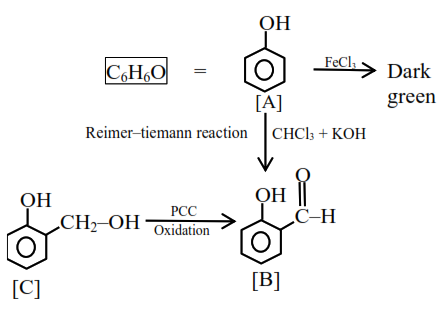# An organic compoundQuestion:

An organic compound $\mathrm{A}\left(\mathrm{C}_{6} \mathrm{H}_{6} \mathrm{O}\right)$ gives dark green colouration with ferric chloride. On treatment with $\mathrm{CHCl}_{3}$ and $\mathrm{KOH}$, followed by acidification gives compound B. Compound B can also be obtained from compound $\mathrm{C}$ on reaction with pyridinium chlorochromate $(\mathrm{PCC}) .$ Identify $\mathrm{A}, \mathrm{B}$ and $\mathrm{C}$.

1.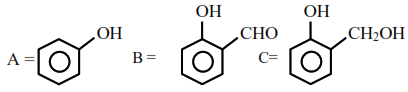2.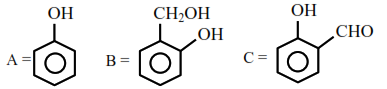3.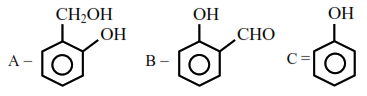4.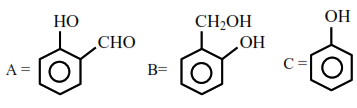Correct Option: 1

Solution: